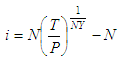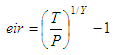# Savings Interest Calculator

The spreadsheet below is a savings interest calculator. It works out nominal and effective interest rates. You have to know your initial investment which amounts to a known total (final or future) value in a specified period of time.

 The Nominal Interest Rate is based on the following formula: The Effective Interest Rate is calculated by the following formula:where:

P = initial investment
T = future value
N = number of compounding periods per year
Y = number of years
i = nominal interest rate
eir = effective interest rate

Note that the number of compounding periods per year is not needed to calculate the effective interest rate.

### Example:

Mr. Hegel invests the modest amount of \$10,000 in a savings bank. Five years later his investment amounts to only \$16,200. What is the effective interest rate offered by his bank? If interest is compounded monthly, what is the nominal interest rate offered?

This is a job for our very useful savings interest calculator... We just have to fill in the blanks...

Initial Investment = 10,000
Final Value = 16,200
Number of years = 5
Nr. of Compounding periods  / yr = 12

and the results are

Nominal Interest rate =     9.687%
Effective Interest rate =     10.129%

 You have to allow JavaScript codes on your navigator, otherwise this calculator won't work.

 Enter the needed values... (do not use commas) Initial Investment: Final Value: Nr. of years: Nr. of compoundig periods per yr: Nominal Interest Rate: % Effective Interest Rate: %

From 'Savings Interest Calculator' to home

From 'Savings Interest Calculator' to Free Online Calculators